NEET  >  Test: Electromagnetic Induction - 2

# Test: Electromagnetic Induction - 2

Test Description

## 25 Questions MCQ Test Physics Class 12 | Test: Electromagnetic Induction - 2

Test: Electromagnetic Induction - 2 for NEET 2023 is part of Physics Class 12 preparation. The Test: Electromagnetic Induction - 2 questions and answers have been prepared according to the NEET exam syllabus.The Test: Electromagnetic Induction - 2 MCQs are made for NEET 2023 Exam. Find important definitions, questions, notes, meanings, examples, exercises, MCQs and online tests for Test: Electromagnetic Induction - 2 below.
Solutions of Test: Electromagnetic Induction - 2 questions in English are available as part of our Physics Class 12 for NEET & Test: Electromagnetic Induction - 2 solutions in Hindi for Physics Class 12 course. Download more important topics, notes, lectures and mock test series for NEET Exam by signing up for free. Attempt Test: Electromagnetic Induction - 2 | 25 questions in 50 minutes | Mock test for NEET preparation | Free important questions MCQ to study Physics Class 12 for NEET Exam | Download free PDF with solutions
 1 Crore+ students have signed up on EduRev. Have you?
Test: Electromagnetic Induction - 2 - Question 1

### A wire loop is placed in a region of time varying magnetic field which is oriented orthogonally to the plane of the loop as shown in the figure. The graph shows the magnetic field variation as the function of time. Assume the positive emf is the one which drives a current in the clockwise direction and seen by the observer in the direction of B. Which of the following graphs best represents the induced emf as a function of time.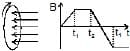Test: Electromagnetic Induction - 2 - Question 2

### A vertical bar magnet is dropped from position on the axis of a fixed metallic coil as shown in fig-I. In fig-II the magnet is fixed and horizontal coil is dropped. The acceleration of the magnet and coil are a1 and a2 respectively then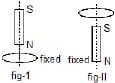Test: Electromagnetic Induction - 2 - Question 3

### An electric current i1 can flow either direction through loop (1) and induced current i2 in loop (2). Positive i1 is when current is from `a' to `b' in loop (1) and positive i2 is when the current is from `c' to `d' in loop (2)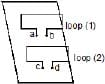In an experiment, the graph of i2 against time `t' is as shown below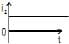Which one(s) of the following graphs could have caused i2 to behave as give above.

Detailed Solution for Test: Electromagnetic Induction - 2 - Question 3

ϕ2=Mi1⇒i2=(1/R)(−dϕ2/dt)
i2=−MdI1/Rdt

Test: Electromagnetic Induction - 2 - Question 4

Figure shows a bar magnet and a long straight wire W, carrying current into the plane of paper. Point P is the point of intersection of axis of magnet and the line of shortest distance between magnet and the wire. If p is the midpoint of the magnet, then which of the following statements is correct ?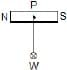Detailed Solution for Test: Electromagnetic Induction - 2 - Question 4

There is a long straight wire W, the current flowing direction in the wire is into the plane of paper and the point of intersection on the axis of magnet is P.
If the midpoint of the bar magnet is P then the distance from the point P to the wire will be the shortest distance.
So, the bar magnet will experience a force along the line of shortest distance.

Test: Electromagnetic Induction - 2 - Question 5

A negative charge is given to a nonconducting loop and the loop is rotated in the plane of paper about its centre as shown in figure. The magnetic field produced by the ring affects a small magnet placed above the ring in the same plane :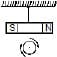*Multiple options can be correct
Test: Electromagnetic Induction - 2 - Question 6

Figure shown plane figure made of a conductor located in a magnetic field along the inward normal to the plane of the figure. The magnetic field starts diminishing. Then the induced current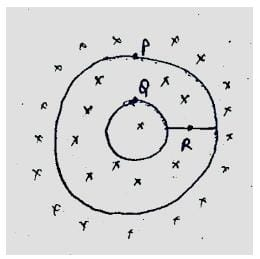Detailed Solution for Test: Electromagnetic Induction - 2 - Question 6As the field diminishes the current is induced in the coils such that if opposes the diminishing of the field. Hence, current is induced in clockwise directing in both the loops.
And as the loops are closed no current will come out of the loops and no current at point R.
Hence option A C D is the correct option.

*Multiple options can be correct
Test: Electromagnetic Induction - 2 - Question 7

A conducting wire frame is placed in a magnetic field which is directed into the paper. The magnetic field is increasing at a constant rate. The directions of induced currents in wires AB and CD are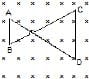Detailed Solution for Test: Electromagnetic Induction - 2 - Question 7

Consider the surface facing upwards having points A, B, C and D in anticlockwise direction when viewing towards the surface from above. Let this be S
flux = S×B
As the flux increases, by Lenz's Law the induced current will be in the direction C B A D C, so as to oppose the increase in flux.

*Multiple options can be correct
Test: Electromagnetic Induction - 2 - Question 8

A bar magnet is moved along the axis of copper ring placed far away from the magnet. Looking from the side of the magnet, an anticlockwise current is found to be induced in the ring. Which of the following may be true?

Detailed Solution for Test: Electromagnetic Induction - 2 - Question 8

When magnet is moving towards the ring, as the current flows in anti-clockwise direction when seen from magnet, a magnetic field directed towards the magnet from the ring is observed.
By Lenz's law this field is to oppose the movement of the magnet towards the ring.
Therefore the N-pole should be facing the ring, as only then will the induced field by the ring will oppose the movement of the approaching magnet.
Therefore, the north pole faces the ring and the magnet moves towards it  and the  south pole faces the ring and the magnet moves away from it.

*Multiple options can be correct
Test: Electromagnetic Induction - 2 - Question 9

Two circular coils P & Q are fixed coaxially & carry currents I1 and Irespectively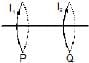*Multiple options can be correct
Test: Electromagnetic Induction - 2 - Question 10

Which of the following quantities can be written in SI units in K gm2 A-2 S-3 ?

Detailed Solution for Test: Electromagnetic Induction - 2 - Question 10

The dimensional formula of a given unit is [ML2T−3A−2].
So, from the given option only resistance has this dimensional formula so resistance is the correct answer.

*Multiple options can be correct
Test: Electromagnetic Induction - 2 - Question 11

AB and CD are smooth parallel rails, separated by a distance l, and inclined to the horizontal at an angle q. A uniform magnetic field of magnitude B, directed vertically upwards, exists in the region. EF is a conductor of mass m, carrying a current i. For EF to be in equilibrium,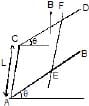Detailed Solution for Test: Electromagnetic Induction - 2 - Question 11

Force on EF,
Fmag​​=i∫(dl×B)=iLBsin(90−θ)=iLBcosθ
Fmag​​ up the inclined plane.
Component of weight of EF down the inclined plane: mgsinθ
For EF to be in equilibrium, iLBcosθ=mgsinθ
∴iLB=mgtanθ
For Fmag​ to be up the inclined plane, it needs to flow from E to F.

*Multiple options can be correct
Test: Electromagnetic Induction - 2 - Question 12

AB and CD are smooth parallel rails, separated by a distance l, and inclined to the horizontal at an angle q. A uniform magnetic field of magnitude B, directed vertically upwards, exists in the region. EF is a conductor of mass m, carrying a current i. if B is normal to the plane of the railsDetailed Solution for Test: Electromagnetic Induction - 2 - Question 12

As seen from the image, in the direction of magnetic field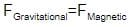mgsinθ=il×B
mgsinθ=ilB

*Multiple options can be correct
Test: Electromagnetic Induction - 2 - Question 13

A conducting rod PQ of length L = 1.0 m is moving with a uniform speed v = 20 m/s in a uniform magnetic field B = 4.0 T directed into the paper. A capacitor of capacity C = 10 mF is connected as shown in figure. Then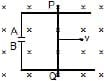Detailed Solution for Test: Electromagnetic Induction - 2 - Question 13

According to Fleming’s right-hand rule induced current flows from Q to P.
Hence P is at higher potential and Q is at lower potential.
Therefore, A is positively charged and B is negatively charged.
Hence:
qA​=+800μC and qb​=−800μC

*Multiple options can be correct
Test: Electromagnetic Induction - 2 - Question 14

two different arrangements in which two square wire frames of same resistance are placed in a uniform constantly decreasing magnetic field B.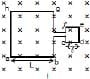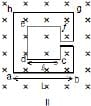The value of magnetic flux in each case is given by

Detailed Solution for Test: Electromagnetic Induction - 2 - Question 14

f=BA, area of square=(side)2
so, for case I.
loops is continuous,
f=(L2+l2)B
for case II
loop is opposite,
so, flux should be subtracted
f=(L2-l2)B

Test: Electromagnetic Induction - 2 - Question 15

Two different arrangements in which two square wire frames of same resistance are placed in a uniform constantly decreasing magnetic field B.The direction of induced current in the case I is

*Multiple options can be correct
Test: Electromagnetic Induction - 2 - Question 16

Two different arrangements in which two square wire frames of same resistance are placed in a uniform constantly decreasing magnetic field B.The direction of induced current in the case II is

Detailed Solution for Test: Electromagnetic Induction - 2 - Question 16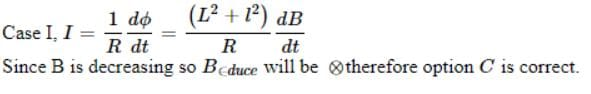*Multiple options can be correct
Test: Electromagnetic Induction - 2 - Question 17

Two different arrangements in which two square wire frames of same resistance are placed in a uniform constantly decreasing magnetic field B.*Multiple options can be correct
Test: Electromagnetic Induction - 2 - Question 18

A conducting ring of radius a is rotated about a point O on its periphery as shown in the figure in a plane perpendicular to uniform magnetic field B which exists everywhere. The rotational velocity is w.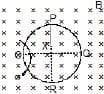Choose the correct statement(s) related to the potential of the points P, Q and R

*Multiple options can be correct
Test: Electromagnetic Induction - 2 - Question 19

A conducting ring of radius a is rotated about a point O on its periphery as shown in the figure in a plane perpendicular to uniform magnetic field B which exists everywhere. The rotational velocity is w.*Multiple options can be correct
Test: Electromagnetic Induction - 2 - Question 20

A conducting ring of radius a is rotated about a point O on its periphery as shown in the figure in a plane perpendicular to uniform magnetic field B which exists everywhere. The rotational velocity is w.Choose the correct statement(s) related to the induced current in ring

Detailed Solution for Test: Electromagnetic Induction - 2 - Question 20

In the given question neither the magnetic field nor the area of the ring is changing. So there will be a constant flux in the ring and hence there will be no EMF (or) current induced in the ring.

*Answer can only contain numeric values
Test: Electromagnetic Induction - 2 - Question 21

The horizontal component of the earth's magnetic field at a place is 3 × 10-4 T and the dip is tan-1(4/3). A metal rod of length 0.25 m placed in the north-south position is moved at a constant speed of 10 cm/s towards the east. Find the e.m.f induced in the rod.

Test: Electromagnetic Induction - 2 - Question 22

A wire forming one cycle of sine curve is moved in x-y plane with velocity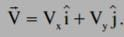. There exist a magnetic field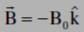. Find the motional emf develop across the ends PQ of wire.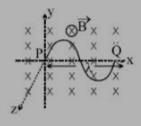*Answer can only contain numeric values
Test: Electromagnetic Induction - 2 - Question 23

A conducting circular loop is placed in a uniform magnetic field of 0.02 T, with its plane perpendicular to the field. If the radius of the loop starts shrinking at a constant rate of 1.0 mm/s, then find the emf induced in the loop, at the instant when the radius is 4 cm.

Detailed Solution for Test: Electromagnetic Induction - 2 - Question 23

Induced EMF E=− (dϕ /dt) ​, ϕ=A.B, A=πR2
this gives, E=−B (dA /dt) ​=−πB×2R (dR/dt) ​
=π×0.02×2×0.04×0.001
=16π×10−7V

Test: Electromagnetic Induction - 2 - Question 24

Find the dimension of the quantity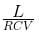, where symbols have usual meaning.

Detailed Solution for Test: Electromagnetic Induction - 2 - Question 24

We know that:
[V]=[iR]=[L (di/dt) ​]
[i][R]= [L][i]​/ [t]
Thus, [L/R​]=[t]=T
[L]= [V][t]​/[i]
Also, i=C (dV/dt) ​, or [CV]=[i][t]=AT
Thus, [L/RCV​]=T/AT=A−1

*Answer can only contain numeric values
Test: Electromagnetic Induction - 2 - Question 25

A rectangular loop with a sliding connector of length l = 1.0 m is situated in a uniform magnetic field B = 2T perpendicular to the plane of loop. Resistance of connector is r = 2W. Two resistances of 6W and 3W are connected as shown in figure. Find the external force required to keep the connector moving with a constant velocity v = 2m/s.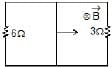Detailed Solution for Test: Electromagnetic Induction - 2 - Question 25

6Ω and 3Ω resistors are in parallel and equivalent resistance is 2Ω+2Ω=4Ω.
F=Bil=BL (​BLv​/Req)
= ​B2l2v/Req ​=(22×12×2​)/4=2N

## Physics Class 12

157 videos|452 docs|213 tests
 Use Code STAYHOME200 and get INR 200 additional OFF Use Coupon Code
Information about Test: Electromagnetic Induction - 2 Page
In this test you can find the Exam questions for Test: Electromagnetic Induction - 2 solved & explained in the simplest way possible. Besides giving Questions and answers for Test: Electromagnetic Induction - 2, EduRev gives you an ample number of Online tests for practice

## Physics Class 12

157 videos|452 docs|213 tests

### How to Prepare for NEET

Read our guide to prepare for NEET which is created by Toppers & the best Teachers